The Scaling Theory of Localization in Experiment, Part II: Carbon Nanotube Electronics

S. L. Hellstrom March 12, 2007

(Submitted as coursework for Applied Physics 272, Stanford University, Winter 2007)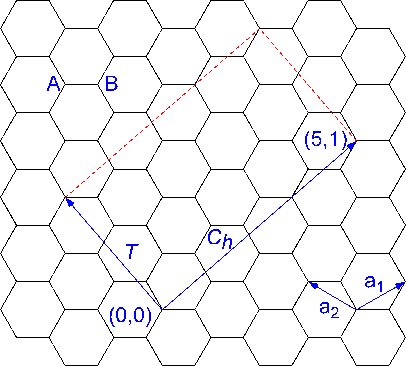Figure 1: Schematic of nanotube structure. a1 and a2 are basis vectors for graphite. Ch is an example of a chiral vector, in this case with (n,m)=(5,1). The corresponding T is (1,3). The area enclosed between these two vectors and the red dotted lines is the unit cell for this sample chiral nanotube. A and B illustrate, as in the text, the sites whose symmetry requires the graphite to have a zero bandgap.  and .

There is a current growing technological interest in systems constrained in one or more dimensions, driven mainly by the push both for fundamentally new devices and for ever-greater integration in the electronics industry. This report provides a brief introduction to carbon nanotubes, recently-discovered allotropes of carbon with unique mechanical and electrical properties, and introduces the first experiment published that purports to measure electrical conductivity in single tubes, as a function of temperature and applied magnetic field. Since many of the modern experiments used to make electrical contact with mesoscopic or molecular conductors use a similar procedure to that presented here, the experimental technique is interesting in its own right. Further since the results of the experiment show behavior consistent with the predictions of the scaling theory of localization, which was introduced in Part I, nanotube electronics are an excellent venue for discussion of this theory in two dimensions and for consideration of some of its predictions for conductance at finite T and in the presence of a magnetic field.

Carbon nanotubes were discovered by Iijima in 1991, after their existence was speculated following the Nobel prize-winning discovery of fullerenes in 1985.1,2 They are, in the simplest sense, rolled-up sheets of graphite; many, called multi-walled carbon nanotubes, actually consist of several concentric layers. Nanotubes are the strongest fibers known to exist and have been applied as a reinforcement in polymers and in concrete.3 Since they are on the order of tens of nanometers in diameter and microns in length, they have a very reliable high aspect ratio and are naturally very sharp. For these properties they have found applications as field-emission electron sources and as tips for scanning probe microscopy.4 Finally, they have very interesting electrical transport characteristics, for their shape makes them naturally effective wires with a high degree of atomic order, and depending on the specific tube structure they may be either metallic or semiconducting.5

The metallic versus semiconducting nature of the carbon nanotube may be explained as follows. Since a carbon nanotube is a finite object, it supports only a discrete set of wave vectors, although in the approximation that the nanotube has infinite length the wave vectors associated with the axial direction become continuous. These discrete wave vectors may be obtained from those of graphite by imposing periodic boundary conditions in the circumferential direction. The energy bands of carbon nanotubes are therefore a set of one-dimensional cross sections of the energy dispersion relations for 2D graphite. Graphite itself is a zero-gap semiconductor; the π and π* energy bands are degenerate at the K and K’ symmetry points. The origin of this degeneracy is the symmetry requirement that the two carbon sites A and B as in Figure 1 are equivalent. So if the energy bands in a given nanotube correspond to a cut through the graphitic K and K’ symmetry points the nanotube will have a band gap of 0, and the nanotube will at this level of analysis be metallic in character. If this is not the case there will be a band gap and the nanotube will be a semiconducting tube.6

The structure of carbon nanotubes is generally described in terms of graphite basis vectors, for example a1 and a2 in Figure 1. From these vectors two are constructed: Ch=na1+ma2, usually abbreviated (n,m), is the vector going completely around the circumference of the nanotube axis, and T is the shortest possible vector along the nanotube axis T=t1a1+t2a2 that connects two lattice sites. The unit cell of the tube in real space is the rectangle formed by these two vectors. These vectors are also illustrated in Figure 1. By a fairly straightforward calculation based on the relevant periodic boundary conditions, it may be shown that a tube is metallic when n-m is a multiple of 3.5

Langer et al. reported the first electrical measurements known of single carbon nanotubes, both with and without an applied magnetic field.7 The nanotubes were made using the carbon arc-discharge method.8 The product of this process was examined via transmission electron microscopy9 and found to contain a predominance of individual nanotubes with average diameter around 18 nm. The nanotubes for which data is reported were multiwalled.

Two of the most critical difficulties currently impeding development of carbon nanotube electronics are 1) inability to selectively grow or separate carbon nanotubes of a particular band structure (e.g., metallic versus semiconducting) and 2) lack of control over location and quality of carbon nanotube-semiconductor substrate interfaces. Langer et. al. ignored the first difficulty – no attempt is made to select for a particular nanotube type. The second difficulty was circumvented by depositing lots of material onto a substrate and then individually selecting appropriate tubes upon which to make measurements. While this is a functional laboratory technique it is clearly not commercially viable. Their process for doing this is shown in Figure 2 and summarized here. The tubes were dispersed onto an oxidized Si wafer with an array of large square gold pads, and an appropriate nanotube identified via microscopy. Then a thin gold film was evaporated, and a thin layer of ω-tricosenic acid (a negative electron photoresist) deposited via the Langmuir-Blodgett technique.10 Gold lines were defined by exposing the photoresist, via scanning tunneling microscopy, between the tube and the nearest gold pads, and the unwanted photoresist and gold film were subsequently removed using ethanol and Ar-ion milling respectively. Alternating-current conductance measurements at 15Hz were performed in a dilution refrigerator in a magnetic field B perpendicular to the tube axis. Four such contacted nanotubes were measured, although the data for only one (with a length of 800 nm and diameter of 20 nm) are given.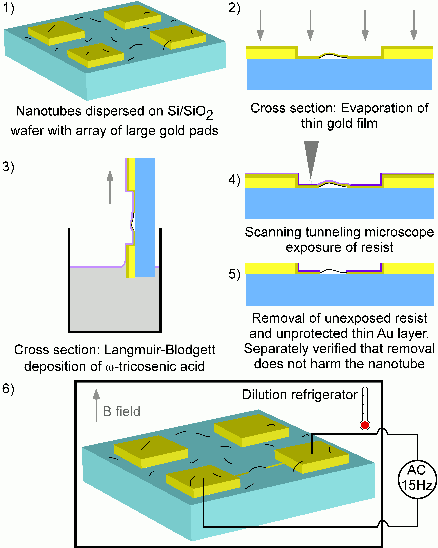Figure 2: Schematic of experiment described in .

Three main results were reported. Firstly, a logarithmic decrease in conductance with decreasing T was noted; this saturated at a T of approximately 0.3K. Secondly, in a magnetic field a positive magnetoconductance (increase in conductance with magnetic field) was observed, and this magnetoconductance existed in some form for all measured T. Finally, reproducible fluctuations of the magnetoconductance with respect to B-field, with amplitude rms[δG]≈9x10-3 e2/h, were noted. A number of these results are explicable using the 2D scaling theory of localization in the weak localization regime as a basis. This in turn implies that in nanotubes elastic scattering is significantly dominant over inelastic scattering, even for fairly high temperatures.

In Part I, it was explained that when Ohm’s law is approximately valid (for large g, where g is the dimensionless conductance), to first order in a perturbation expansion β(g)=(d-2) – (a/g), where β(g) is the scaling parameter, positive for systems with extended electron wavefunctions and negative for systems with localized electron wavefunctions. d is the dimensionality, a is a constant, L is the length of the sample and g is the dimensionless conductance of the system. In two dimensions, β(g)=-a/g. Since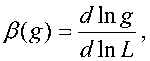by integrating between length scales l and L, where l is the mean free path of the system, one obtains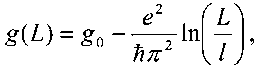where g0 is the conductance of the system at scale l.11

It may be argued that the dependence on temperature in the weakly localized 2D system is of the same form as the L-dependence, down to some cutoff temperature. This argument is justified by the identification of a temperature-dependent length that scales inversely with temperature: LT(T) is proportional to T-p/2, with p some system-dependent index. Since temperature causes random fluctuations in the time evolution of an electronic state, the electron will only diffuse a certain length, even in a perfect crystal, before losing phase coherence. 11 For some T>T0 this length will become smaller than l and will become the characteristic system length. One may then justifiably replace L/l in the above equation with (T/T0)-p/2 so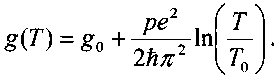This is precisely what is seen in the experiments by Langer et. al., at zero magnetic field, after correcting for the presence of n concentric 2D cylinders, as shown in Figure 3. The data fits well for p=1 and n=4.7

Extending the results of weak localization theory to account for behavior in the presence of a magnetic field involves modification of the perturbation theory correction to β. Since the origin of the correction is interference between a scattered electron traveling in a self-crossing path (one that makes a loop) and one traveling the time-reversed path, i.e., the same path in the opposite direction, any modification to the system that breaks time-reversal invariance will affect this interference. A uniform magnetic field breaks such symmetry, since there is flux going through the loop in a particular direction, and electrons traversing a self-crossed path in opposite directions will acquire different phases. The effects of the interference will be abated for magnetic fields larger than that required for the Landau orbit size (2πeH/hc)1/2 to become smaller than the phase coherence length of the H=0 system. Since the effect of the magnetic field is to reduce the effect of the negative correction to β, it promotes increasingly extended electronic states and higher conductance. This then explains the positive magnetoconductance observed by Langer. More quantitative analyses of this effect may be found in  and .

The above reasoning implies that for a range of magnetic fields there should be some sufficiently high temperature that the temperature-induced phase coherence length is smaller than the smallest relevant Landau orbit size. At this temperature the conductance should become independent of magnetic field. In contrast, Langer et. al. observe positive magnetoconductance at all T measured. They attribute this to an increase in the density of states around the Fermi level in both semiconducting and metallic tubes, a result of the addition of Landau levels to the band structure with the presence of a magnetic field.5 The increase in the density of states also serves to increase the system conductance, irrespective of temperature.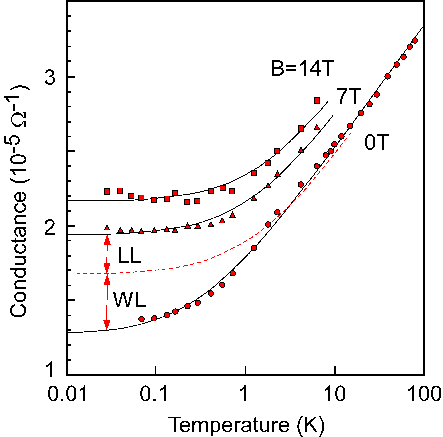Figure 3: Conductance as a function of temperature and magnetic field in a multiwalled carbon nanotube. Solid lines are fits with results from 2D weak localization theory assuming the effect of n cylinders causes G = (σπnd)/L. Fit parameters are p=1, n=4, and T0 = 0.3, 1.1 and 1.5K at B = 0, 7, and 14T respectively. The dashed line shows the differing contributions to the magnetoconductance of weak localization and the Landau levels. 

Finally, the reproducible, aperiodic fluctuations in conductance with variation in magnetic field are examples of universal conductance fluctuations, which had been observed previously in small wires and rings.13,14 Physically, as in the case of the weak localization corrections to β, they originate in interference between different classical electron trajectories. However, this interference depends on the exact impurity structure of a particular sample. In general phases associated with different trajectories have an arbitrary relationship; their interference will result in a particular conductance for a particular impurity configuration. Changing the impurity configuration results in chaotic variation of the interference. The root mean square amplitude variations in conductance associated with these changes in interference are of a magnitude comparable to the relative variance of g, and are predicted to be approximately 0.862 for a 2D system.15 This means that fluctuations in measured sample conductance should be of the order e2/h.

Rather than changing the impurity configuration of a sample to see these fluctuations, one may simply apply a magnetic field. The field imposes an additional arbitrary relative phase to each pair of paths, because different trajectories enclose different amounts of flux. So, the paths will interfere differently as if the impurity configuration had changed. Such conductance fluctuations are observable in systems that are small enough and cold enough that some phase coherence is maintained across the sample; if the sample is hot enough or localized enough that phase coherence across the sample is not maintained these fluctuations will ensemble average to zero.16 The fluctuations observed by Langer et. al. have an rms amplitude that is two orders of magnitude lower than might be expected. They attribute this difference to statistical self-averaging of the fluctuations of different conductors in series and in parallel, since in the measured carbon nanotubes the phase coherence length is smaller than, although on the order of, the tube dimensions.

In sum, the scaling theory of localization, particularly in the weak localization limit, is very useful in explaining the results of the first conductance measurements in carbon nanotubes, and will likely continue to be of great importance in interpretation of results from an ever-increasing number of mesoscopic electronics experiments.

© 2007 S.L. Hellstrom. The author grants permission to copy, distribute and display this work in unaltered form, with attribution to the author, for noncommercial purposes only. All other rights, including commercial rights, are reserved to the author.

References

 S. Iijima, "Helical Microtubules of Graphitic Carbon," Nature 354, 56 (1991).

 H.W. Kroto , "C60: Buckminsterfullerene," Nature 318, 162 (1985).

 E.T. Thostenson and T.-S. Chou, "On the Elastic Properties of Carbon Nanotube-Based Composites: Modelling and Characterization," J. Phys. D 36, 573 (2003).

 H. Dai et al., "Nanotubes as Nanoprobes in Scanning Probe Microscopy," Nature 384, 147 (1996).

 H. Ajiki and T. Ando, "Electronic States of Carbon Nanotubes," J. Phys. Soc. Jpn. 62, 1255 (1993).

 R. Saito, G. Dresselhaus and M.S. Dresselhaus, Physical Properties of Carbon Nanotubes, (Imperial College Press, 1998).

 L. Langer et al. "Quantum Transport in a Multiwalled Carbon Nanotube," Phys. Rev. Lett. 76, 479 (1996).

 T.W. Ebbesen and P.M. Ajayan, "Large-Scale Synthesis of Carbon Nanotubes," Nature 358, 220 (1992).

 S.C. Tsang et al, "The Structure of the Carbon Nanotube and its Surface Topography Probed by Transmission Electron Microscopy and Atomic Force Microscopy," Chem. Phys. Lett. 249, 413 (1996).

 I.R. Peterson, "Langmuir-Blodgett Films," J. Phys. D 23, 379 (1990).

 P.A. Lee and T.V. Ramakrishnan, "Disordered Electronic Systems," Rev. Modern Phys. 57, 287 (1985).

 B.L. Altshuler, D. Khmel'nitzkii, A.I. Larkin, P.A. Lee, "Magnetoresistance and Hall Effect in a Disordered Two-Dimension Electron Gas," Phys. Rev. B. 22, 5142 (1980).

 C.P. Umbach, S. Washburn, R.B. Laibowitz and R.A. Webb, "Magnetoresistance of Small, Quasi-One-Dimensional, Normal-Metal Rings and Lines," Phys. Rev. B, 1984, 30, 4048.

 A.B. Fowler, A. Hartstein and R.A. Webb, "Conductance in Restricted-Dimensionality Accumulation Layers," Phys. Rev. Lett. 48, 196 (1982).

 P.A. Lee and A.D. Stone, "Universal Conductance Fluctuations in Metals," Phys. Rev. Lett. 55, 1622 (1985).

 P.A. Lee, A.D. Stone and H. Fukuyama, "Universal Conductance Fluctuations in Metals: Effects of Finite Temperature, Interactions, and Magnetic Field," Phys. Rev. B. 35, 1039 (1987).